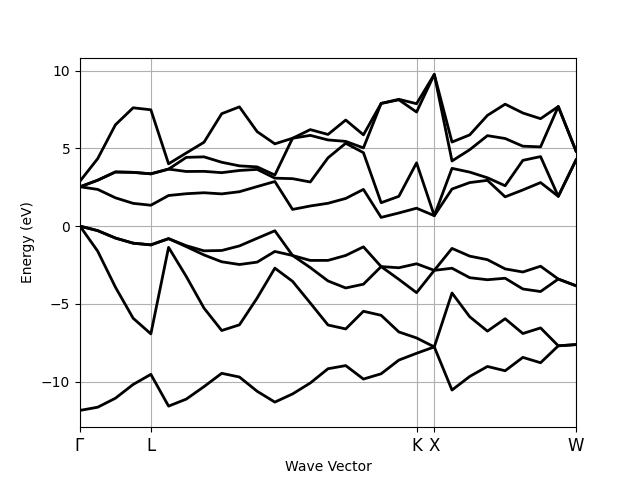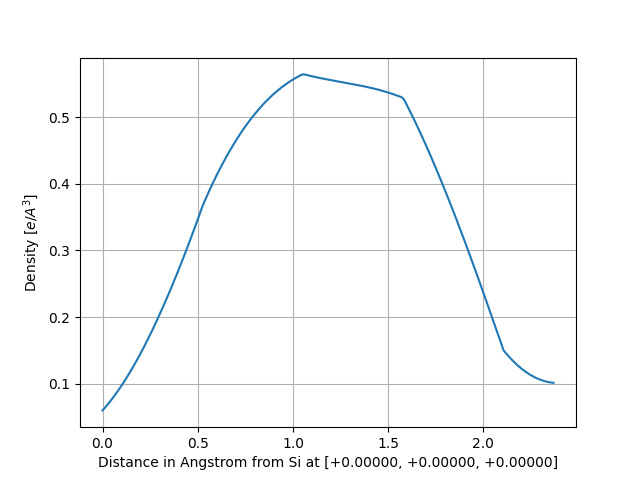# Density¶

This example shows how to analyze the electronic density stored in the DEN.nc file.

•••Out:

Full Formula (Si2)
Reduced Formula: Si
abc   :   3.866975   3.866975   3.866975
angles:  60.000000  60.000000  60.000000
Sites (2)
#  SP       a     b     c
---  ----  ----  ----  ----
0  Si    0     0     0
1  Si    0.25  0.25  0.25

Abinit Spacegroup: spgid: 227, num_spatial_symmetries: 48, has_timerev: True, symmorphic: True
Density: nspinor: 1, nsppol: 1, nspden: 1
Mesh3D: nx=18, ny=18, nz=18
/Users/gmatteo/git_repos/pymatgen/pymatgen/util/plotting.py:550: UserWarning: Matplotlib is currently using agg, which is a non-GUI backend, so cannot show the figure.
plt.show()
/Users/gmatteo/git_repos/pymatgen/pymatgen/util/plotting.py:550: UserWarning: Matplotlib is currently using agg, which is a non-GUI backend, so cannot show the figure.
plt.show()
/Users/gmatteo/git_repos/abipy/abipy/core/fields.py:413: DeprecationWarning: get_neighbors_old is deprecated; use get_neighbors in pymatgen.core.structure instead.
This is retained purely for checking purposes.
/Users/gmatteo/git_repos/pymatgen/pymatgen/util/plotting.py:550: UserWarning: Matplotlib is currently using agg, which is a non-GUI backend, so cannot show the figure.
plt.show()


from abipy.abilab import abiopen
import abipy.data as abidata

# Open the DEN.nc file
ncfile = abiopen(abidata.ref_file("si_DEN.nc"))

# The DEN file has a Density, a Structure and an ElectronBands object
print(ncfile.structure)

# To plot the KS eigenvalues.
#ncfile.ebands.plot()

density = ncfile.density
print(density)

# To visualize the total charge wih vesta
#visu = density.visualize("vesta"); visu()

# To plot the density along the line connecting
# the first and the second in the structure:
density.plot_line(point1=0, point2=1)

# alternatively, one can define the line in terms of two points
# in fractional coordinates:
density.plot_line(point1=[0, 0, 0], point2=[2.25, 2.25, 2.25], num=300)

# To plot the density along the lines connect the firt atom in the structure
# and all the neighbors within a sphere of radius 3 Angstrom: